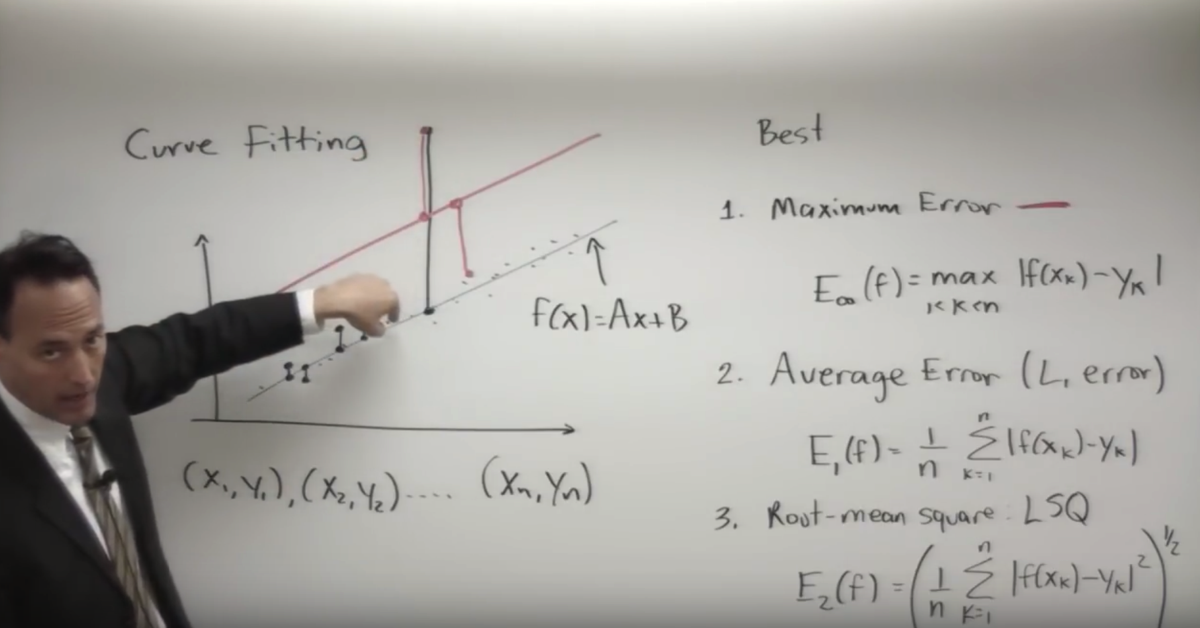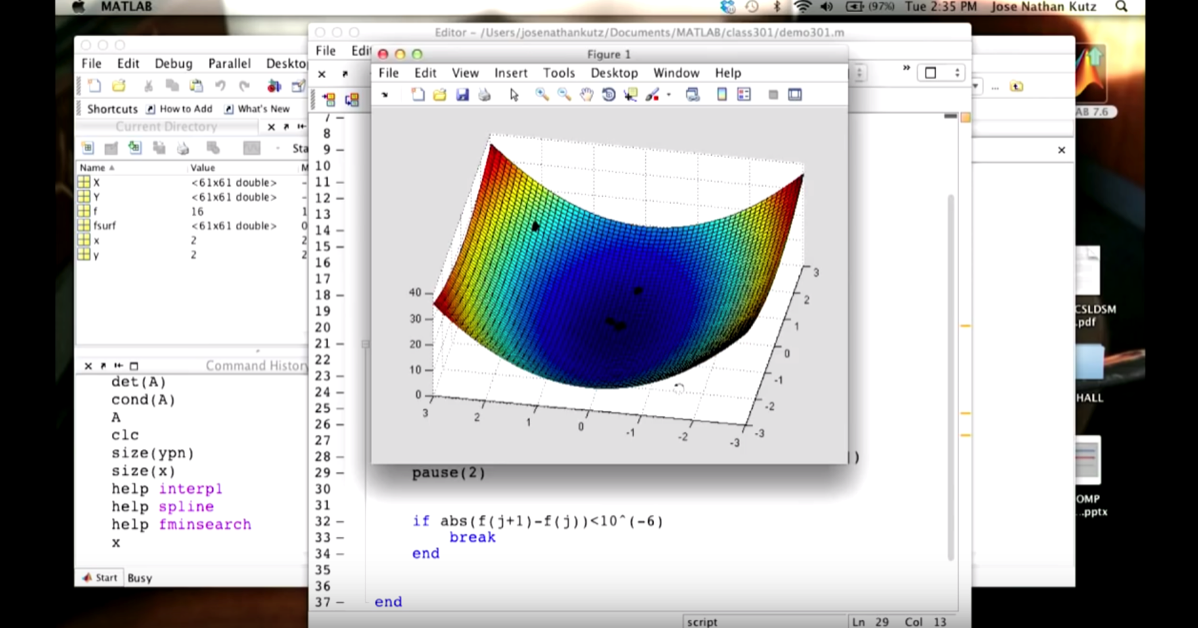Machine Learning, Dynamical Systems and Control

All of machine learning revolves around optimization. This includes regression and model selection frameworks that aim to provide parsimonious and interpretable models for data. Curve fitting is the most basic of regression techniques, with polynomial and exponential fitting resulting in solutions that come from solving linear systems of equations. This can be generalized for fitting of nonlinear systems. Importantly, regression are typically applied to under- or over-determined systems, thus requiring cross-validation strategies to evaluate the results.

Section 4.1: Least-Squares RegressionSection 4.2: Gradient Descent Algorithm